Technical Article

February 05, 2018 by Robert Keim

## This article, part of AAC’s Analog Circuit Collection, presents a straightforward way to precisely control the current through an LED (or through a typical resistive load).

This article, part of AAC’s Analog Circuit Collection, presents a straightforward way to precisely control the current through an LED (or through a typical resistive load).

As you have probably noticed, real-life electronic design tends to exhibit a certain dominance of voltage over current. What I mean is the following: Stable voltage sources are common and widely available, whereas it takes some effort to create anything approaching the ideal current sources that appear in theoretical circuit diagrams. Consequently, the current flowing through a load is generally determined by the amplitude of the applied voltage and the load’s current–voltage characteristics. In the case of an ordinary resistive load, the current–voltage relationship is simply the resistance. Thus, current equals voltage divided by resistance. If the load resistance changes, the load current changes proportionally.

This is usually fine, but there are situations in which we want to directly specify the current, regardless of the characteristics of the load. In such cases we can use a voltage-to-current converter (VCC), which is essentially a voltage-controlled current source. We generate a voltage signal according to our typical methods, then we use the VCC to generate a current that is dependent only on this input voltage.

### Applications

I can’t think of too many situations in which you would need to use a VCC to carefully control the current through a resistive load. Actually, at the moment I can’t think of any (feel free to leave a comment if you can help me out here...). The linear nature of a resistor’s current–voltage relationship makes the current-to-voltage conversion somewhat redundant: (directly) increasing the voltage has, in general, the same effect as using a voltage to increase the current.

There is no doubt, however, that a VCC is a very handy circuit when you are working with light-emitting diodes. An LED (being a diode) has nonlinear current-voltage characteristics, and since the amount of light energy generated by the LED is determined by the current flowing through it, voltage is by no means a straightforward way to control brightness.

This issue is discussed in this technical brief and also in this project article. If you read through the project, you’ll see that I used a simple op-amp circuit as a voltage-to-current converter: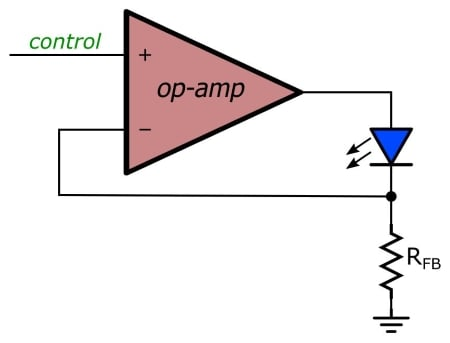Actually, I used three such circuits to create a “single-pixel” color display using an RGB LED. In this article I’ll show you a different circuit that accomplishes the same thing. How do they compare? Well, the previous circuit uses only one op-amp per diode, whereas the new one uses two, but op-amps are so tiny these days that this can hardly be considered a significant advantage. Actually, a one-op-amp circuit can even be a bit of a nuisance when you already have a dual-op-amp IC, because you’re left with the surprisingly nontrivial task of neutralizing the unused amplifier. I wouldn’t say that one of these circuits is strictly better than the other, but there is one important difference that I’ll explain in the next section.

### Analysis

Here is the circuit: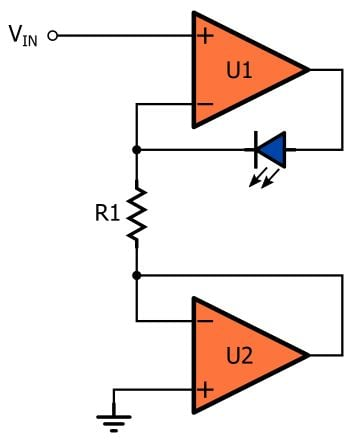Let’s consider the functionality before we look at the simulation plots. The circuit consists more or less of two interconnected voltage followers; I say “more or less” because one of the followers has a diode in the feedback path. The follower on the bottom has ground as its input voltage, so the output voltage, and therefore also the voltage at the inverting input terminal, is 0 V. This means that the lower terminal of R1 will always be at 0 V.

We know from the “virtual short” approximation that an op-amp’s two input terminals will be at the same voltage. This means that the voltage at the upper terminal of R1 is equal to the control voltage, and thus the current through R1 is always VCONTROL/R1. But what is the source of this current? It certainly can’t be sourced by U1’s inverting input terminal, and the only other possibility is U1’s output terminal. Thus, the current flowing from U1’s output is always VCONTROL/R1, and consequently the current through the diode is always VCONTROL/R1. The same would apply if the diode were replaced by a resistor, or even an inductor. In other words, this circuit is used to precisely control the current flowing through the component that is placed in U1’s feedback path.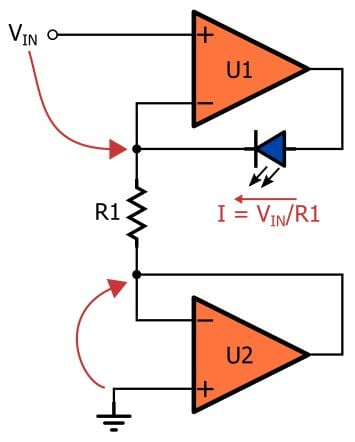The primary difference between this circuit and the one-op-amp-circuit used in the single-pixel-color-display project is the following: the two-op-amp circuit is differential, whereas the one-op-amp circuit is single-ended. In the one-op-amp circuit, the input is always referenced to ground. The dual-op-amp configuration allows you to control the load current using a differential voltage; this is depicted in the following circuit diagram, where U2’s noninverting terminal has become a second input rather than a direct connection to ground.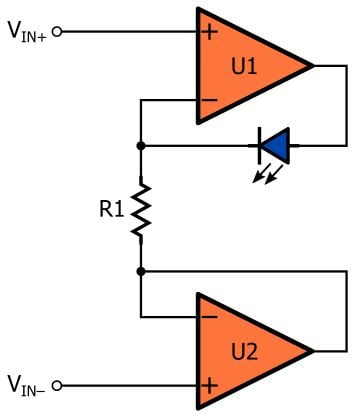Here’s the LTspice implementation, followed by two plots. In the first, you can see only one trace because the two traces (input voltage and load current) are perfectly overlapping. In the second plot I put the two traces in separate panes. These plots confirm that the load current carefully follows the input voltage, despite the diode’s complicated current–voltage characteristics, and that the relationship between input voltage and load current is indeed the (pleasantly simple) equation explained above, i.e., IL = VIN/R1.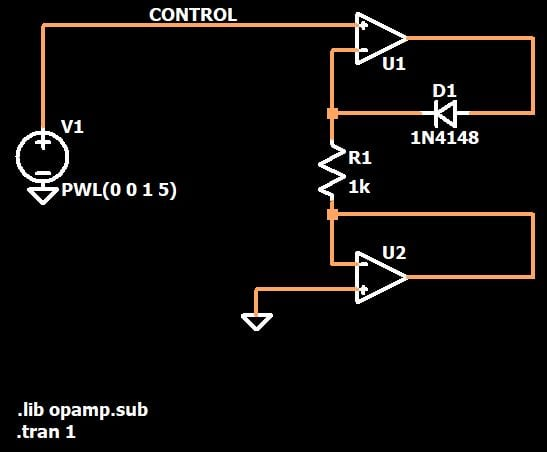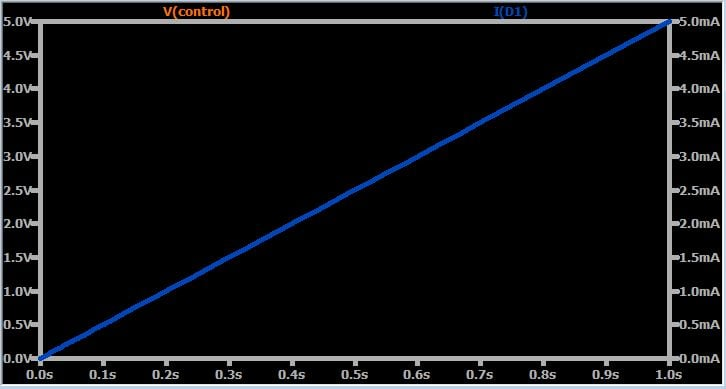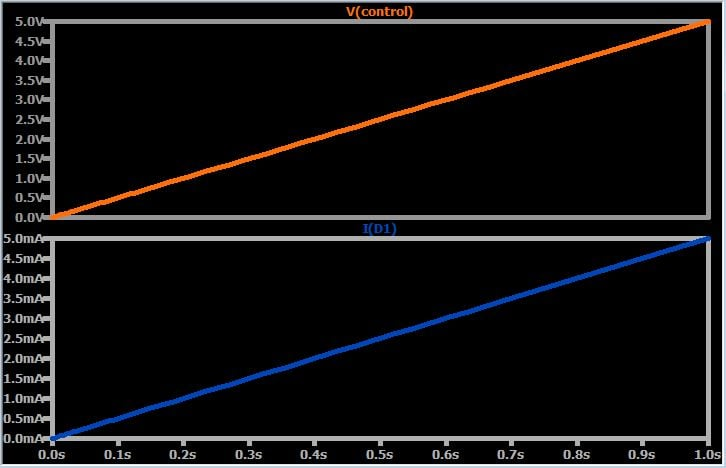### Conclusion

The article has presented and explained a simple yet effective circuit for using a voltage signal to precisely control the current through a load component. This configuration is more versatile than a circuit that I used previously because it accepts differential input voltages. However, remember that not every op-amp has rail-to-rail inputs; if the negative supply is at 0 V, some op-amps will not be compatible with the grounded-input version of the VCC discussed in this article.

If you want to save yourself a little bit of work, you can download my LTspice schematic by clicking on the orange button.

VCC.zip•zhong wang February 08, 2018

good article

Like.
• W
whitehaired novice February 08, 2018

I found this very interesting.  Can you recommend a good op amp to use in your circuit with current range of 10 mA and less?  whitehaired novice

Like.
•RK37 February 17, 2018
Just about any general-purpose op-amp should be sufficient for a typical voltage-to-current converter such as this one. However, you do have to make sure that the op-amp can supply enough current for your LED. My recommendation is to use a distributor search tool (I use Digi-Key) and look for general-purpose op-amps with an adequate output-current rating.
Like.